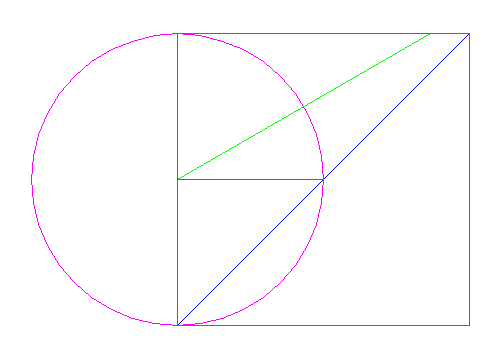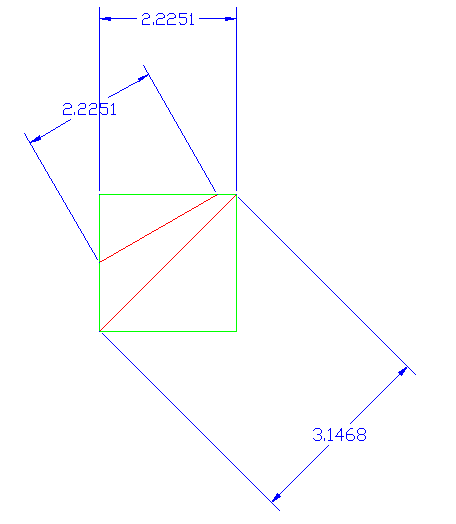Previous-------Page 1 2------- Next-------Math Home -------   The following pages of “Hunches” are presented for the possibility of creating new ideas. They are also interesting to see how the problem was approached. However, many things presented here though they may show creative thought are mathematically incorrect. Repeated these exercises are for ideas and contain many logic and mathematical mistakes. They are still interesting especially if the reader is looking for new problems to solve, getting ideas or brainstorming. That is why this section is recorded here.

_______
20060103
On a circle 2 different angles will yield the same sine (y component value) if spaced a certain given distance, a length away from the origin. This is not new.

But for two angles 30 degrees and 45 degrees, their lengths may differ but if the 30 degree line Is placed at the origin and the and the 45 degree angle is placed 1 radian or r away from the origin on the x-axis the lines will meet at a height of r (from the origin) and
sin(45) * r = (sin (30)* r) + r

It would be interesting to see what this looked liked graphed on a unit circle. (Combine radians and degrees.)

½ (cos(30) * r) = sin(45) * r

Need to find relationship (=) between angles._______
20060309

(Diameter / cos (45) ) - r

r / cos(45) - r = ???

(D * sin(45) ) - r = sin(30) *r

still can’t describe it, but the idea is thereLet’s look at a square the circumscribes the circle that we need values from. In this example the square’s length and width are 2.225125 half the size as the arched door problem, but the same applies to any square. 2.22512 * 2 = 4.45024 r + r = D 4.45024 * sin(45) = 3.14679488 D * sin 45 = r / sin 45 is the length of the bisector of the square 3.14679488 - 2.225125 = 0.92167 bisector - r = difference of 45 length - 30 length 3.1468 - 0.92167 = 2.225125 which is the length of the 30 degree angle segment Need equation though D * sin(45) - (D * 45 - r) = length of r = sin(30) * D